# Problem-1 (Materials, labor and variable overhead variances)

P&G company produces many products for household use. Company sells products to storekeepers as well as to customers. Detergent-DX is one of the products of P&G. It is a cleaning product that is produced, packed in large boxes and then sold to customers and storekeepers.

P&G uses a traditional standard costing system to control costs and has established the following materials, labor and overhead standards to produce one box of Detergent-DX:

• Direct materials; 1.5 pounds @ \$12 per pound: \$18.00
• Direct labor; 0.6 hours \$24 per hour: \$14.40
• Variable manufacturing overhead; 0.6 hours @ \$5.00: \$3.00

During August 2012, company produced and sold 3,000 boxes of Detergent-DX. 8,000 pounds of direct materials were purchased @ \$11.50 per pound. Out of these 8,000 pounds, 6,000 pounds were used during August. There was no inventory at the beginning of August. 1600 direct labor hours were recorded during the month at a cost of \$40,000. The variable manufacturing overhead costs during August totaled \$7,200.

Required:

1. Compute materials price variance and materials quantity variance. (Assume that the materials price variance is computed at the time of purchase.)
2. Compute direct labor rate variance and direct labor efficiency variance.

## Solution:

### (1). Materials variances:

a. Materials price variance: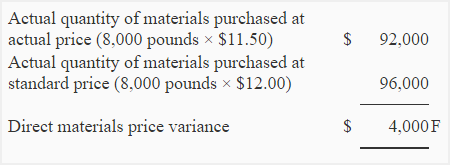b. Materials quantity variance: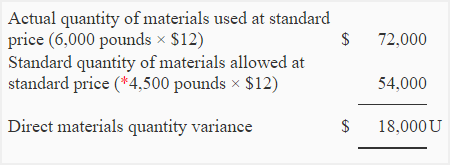*3,000 boxes × 1.5 pounds per box = 4,500 pounds

F = Favorable; U = Unfavorable

### (2). Labor variances:

a. Direct labor rate variance: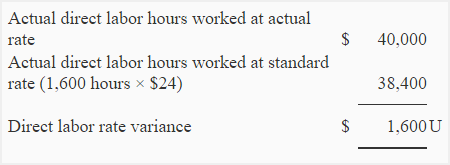b. Direct labor efficiency variance:*3,000 boxes × 0.6 hours per box = 1,800 hours

F = Favorable; U = Unfavorable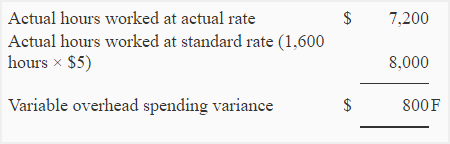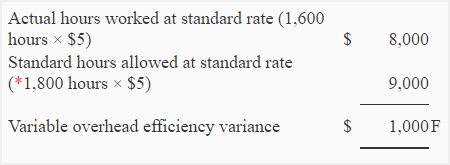*3,000 boxes × 0.6 hours per box = 1,800 hours

F = Favorable

A D V E R T I S E M E N T
1.zeke

In number 1 solution, where didi you get the 4,500 standard quantity of materials allowed?

1.Issack

It’s the quantity of material produced. 3000 boxes * 1.5 pounds per box = 4500 pounds of material

2.Accounting For Management

@zeke
Standard quantity of material per unit × number of units produced
= 1.5 × 3000
= 45,000

3.Riley

Hi for number 2 direct labor efficiency, where did you get the 1,800 hours?

4.Accounting For Management

@Riley

Direct labor hours allowed for actual production:
Actual units produced × standard hours per unit
3,000 units × 0.6 hours
1,800 hours

5.sumair

where did 4.5 accur in variable variance as actual rate

6.Accounting For Management

@Sumair
Actual variable manufacturing overhead costs/actual hours worked
\$7,200/1600 hours
\$4.50

7.Jai

please can you explain how you got \$5 in the variable overhead spending variance?

1.Accounting For Management

It is standard variable overhead rate per hour (given in the problem).

8.bony

what was the labour ,material and over head cost for water pollution control

9.tracy

thank you so much um sure i have understood

10.margret gwanyanya

you helped me ooooo

11.sir. i m glad if you send me all question with solutions of all kind of variance. thanks.

12.reina

Im helped thanks a lot

13.noman

in material quantity variance
where does this “3000 boxes” comes from

1.Accounting For Management

Did you read the problem text? Company produced and sold 3,000 boxes of detergent DX during August.

14.Ralex Markis

Hi,

I have an exercise on standard costing and variance.

ABC Ltd prepares the following master budget for 2016
Sales (18,000 units @ \$30) \$540,000
Materials (9,000 kg @ \$10) \$ 90,000
Labour (36,000 hours @ \$6) \$216,000
VOH (36,000 @\$2) \$ 72,000
FOH \$ 90,000
Budgeted Profit \$ 72,000

Their trial balance at 31st December 2016 reveals the following figures;
Sales (20,000 units @ \$30) \$600,000
Materials (10,500 kg) \$ 99,750
Labour @ \$6.25/hour \$243,750
VOH \$ 76,050
FOH \$ 87,000
Gross Profit \$ 93,450

How do I get the following;
1. Material Price Variance,
2. Material Usage Variance,
3. Labour Rate Variance,
4. Labour Efficiency Variance,
5. VOH Rate Variance,
6. VOH Efficiency Variance &
7. FOH Budget Variance.

Grateful if you can show me step by step on getting to the solution.

Many thanks & regards,
Ms. Ralex Markis

1.XYZ

material puchased- 24000 [email protected]
Material consumed- 22800kgs
actual wages paid for 5940 hrs- Rs. 29700
units produced- 2160
direct material cost- Rs. 4per unit
direct labour cost- 4 per unit
standard input- 10kg for one unit
calculate the material variance & labour variance
how can i solve the question

1.pk

is it solved…

15.zaman nagra

sir plz send all variance and management accounting paper of perpetration icma

16.Hafsa

Why do you compute 4500 pounds in 1(b)

1.Accounting For Management

Because it is required component for the computation of materials quantity variance. The formula of this variance is:
(Actual quantity used x Standard price) – (Standard quantity allowed x Standard price)
The standard quantity allowed for producing 3,000 boxes is not available in the original problem so we have to compute it as follows:
Actual production x Standard quantity per unit
= 3,000 boxes x 1.5 pounds
= 4,500 pounds
Hope that helps 🙂